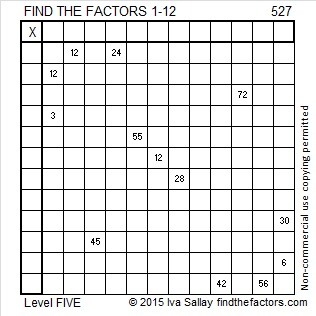# 527 and Level 5

527 is the sum of consecutive primes including all the prime numbers in its prime factorization. Can you list those consecutive primes?Print the puzzles or type the solution on this excel file: 12 Factors 2015-06-15

—————————————————————————————————

• 527 is a composite number.
• Prime factorization: 527 = 17 x 31
• The exponents in the prime factorization are 1 and 1. Adding one to each and multiplying we get (1 + 1)(1 + 1) = 2 x 2 = 4. Therefore 527 has exactly 4 factors.
• Factors of 527: 1, 17, 31, 527
• Factor pairs: 527 = 1 x 527 or 17 x 31
• 527 has no square factors that allow its square root to be simplified. √527 ≈ 22.95648—————————————————————————————————This site uses Akismet to reduce spam. Learn how your comment data is processed.